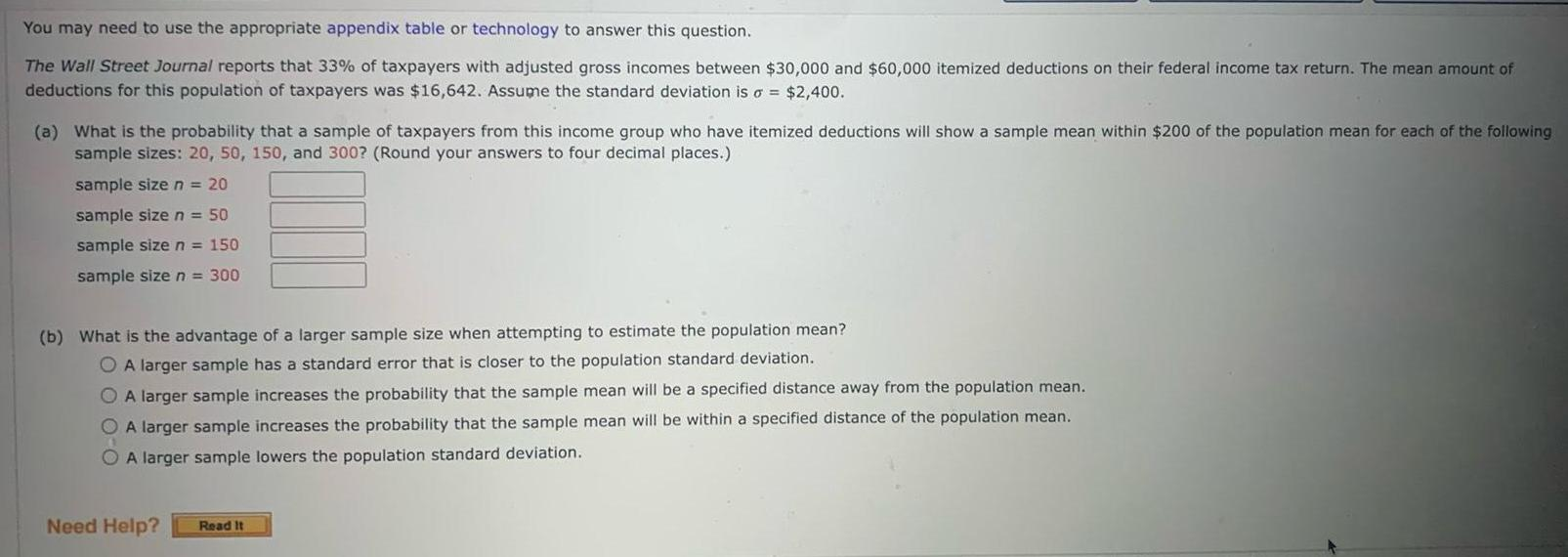Question:

# You may need to use the appropriate appendix table or

Last updated: 11/21/2023You may need to use the appropriate appendix table or technology to answer this question The Wall Street Journal reports that 33 of taxpayers with adjusted gross incomes between 30 000 and 60 000 itemized deductions on their federal income tax return The mean amount of deductions for this population of taxpayers was 16 642 Assume the standard deviation is o 2 400 a What is the probability that a sample of taxpayers from this income group who have itemized deductions will show a sample mean within 200 of the population mean for each of the following sample sizes 20 50 150 and 300 Round your answers to four decimal places sample size n 20 sample size n 50 sample size n 150 sample size n 300 b What is the advantage of a larger sample size when attempting to estimate the population mean A larger sample has a standard error that is closer to the population standard deviation OA larger sample increases the probability that the sample mean will be a specified distance away from the population mean O A larger sample increases the probability that the sample mean will be within a specified distance of the population mean A larger sample lowers the population standard deviation Need Help Read It